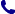• Kontakt
• Newsletter Zapisz się po informacje o nowościach i specjalnych ofertach. Administrator danych: ABE-IPS sp. z o.o.
Wybierz interesujące Cię kategorie
Wyrażam zgodę na przetwarzanie moich danych osobowych w celach marketingowych, tym samym akceptuję Regulamin Newslettera i Politykę prywatności.
Wyrażam zgodę na przesyłanie informacji handlowych drogą elektroniczną.
Wypisz się
• Klienci instytucjonalni
• Dotacje UE
• O nas
Dostęp on-line

# Książki

0.00 PLN
Schowek (0)
 Schowek jest pusty

## Algebra

 Autorzy Wydawnictwo Birkhauser Boston Inc Data wydania 09/07/2003 Liczba stron 160 Forma publikacji książka w miękkiej oprawie Poziom zaawansowania Dla szkół wyższych i kształcenia podyplomowego ISBN 9780817636777 Kategorie Algebra
Zapytaj o ten produkt
 E-mail Pytanie Wyślij
Do schowka

## Opis książki

This book is about algebra. This is a very old science and its gems have lost their charm for us through everyday use. We have tried in this book to refresh them for you. The main part of the book is made up of problems. The best way to deal with them is: Solve the problem by yourself - compare your solution with the solution in the book (if it exists) - go to the next problem. However, if you have difficulties solving a problem (and some of them are quite difficult), you may read the hint or start to read the solution. If there is no solution in the book for some problem, you may skip it (it is not heavily used in the sequel) and return to it later. The book is divided into sections devoted to different topics. Some of them are very short, others are rather long. Of course, you know arithmetic pretty well. However, we shall go through it once more, starting with easy things. 2 Exchange of terms in addition Let's add 3 and 5: 3+5=8. And now change the order: 5+3=8. We get the same result. Adding three apples to five apples is the same as adding five apples to three - apples do not disappear and we get eight of them in both cases. 3 Exchange of terms in multiplication Multiplication has a similar property. But let us first agree on notation.

Algebra

## Spis treści

1 Introduction.- 2 Exchange of terms in addition.- 3 Exchange of terms in multiplication.- 4 Addition in the decimal number system.- 5 The multiplication table and the multiplication algorithm.- 6 The division algorithm.- 7 The binary system.- 8 The commutative law.- 9 The associative law.- 10 The use of parentheses.- 11 The distributive law.- 12 Letters in algebra.- 13 The addition of negative numbers.- 14 The multiplication of negative numbers.- 15 Dealing with fractions.- 16 Powers.- 17 Big numbers around us.- 18 Negative powers.- 19 Small numbers around us.- 20 How to multiply am by an, or why our definition is convenient.- 21 The rule of multiplication for powers.- 22 Formula for short multiplication: The square of a sum.- 23 How to explain the square of the sum formula to our younger brother or sister.- 24 The difference of squares.- 25 The cube of the sum formula.- 26 The formula for (a + b)4.- 27 Formulas for (a + b)5, (a + b)6,... and Pascal's triangle.- 28 Polynomials.- 29 A digression: When are polynomials equal?.- 30 How many monomials do we get?.- 31 Coefficients and values.- 32 Factoring.- 33 Rational expressions.- 34 Converting a rational expression into the quotient of two polynomials.- 35 Polynomial and rational fractions in one variable.- 36 Division of polynomials in one variable; the remainder.- 37 The remainder when dividing by x - a.- 38 Values of polynomials, and interpolation.- 39 Arithmetic progressions.- 40 The sum of an arithmetic progression.- 41 Geometric progressions.- 42 The sum of a geometric progression.- 43 Different problems about progressions.- 44 The well-tempered clavier.- 45 The sum of an infinite geometric progression.- 46 Equations.- 47 A short glossary.- 48 Quadratic equations.- 49 The case p =. Square roots.- 50 Rules for square roots.- 51 The equation x2 + px + q =.- 52 Vieta's theorem.- 53 Factoring ax2 + bx + c.- 54 A formula for ax2 + bx + c = (where a ? 0).- 55 One more formula concerning quadratic equations.- 56 A quadratic equation becomes linear.- 57 The graph of the quadratic polynomial.- 58 Quadratic inequalities.- 59 Maximum and minimum values of a qua ratic polynomial.- 60 Biquadratic equations.- 61 Symmetric equations.- 62 How to confuse students on an exam.- 63 Roots.- 64 Non-integer powers.- 65 Proving inequalities.- 66 Arithmetic and geometric means.- 67 The geometric mean does not exceed the arithmetic mean.- 68 Problems about maximum and minimum.- 69 Geometric illustrations.- 70 The arithmetic and geometric means of everal numbers.- 71 The quadratic mean.- 72 The harmonic mean.

## Polecamy również książki801 777 223